## Personal Finance Big Ideas taught in this task: Inflation

Inflation is the measure of the annual growth in the price of a good or service, computed as the percentage change in price from the previous year. For example, if the price of gasoline rose from \$2.00 a gallon to \$2.20 per gallon in one year, the inflation rate for gasoline for that year would be: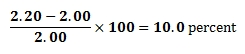1. Suppose in the following year, the price of gasoline rose another twenty cents per gallon to \$2.40. Find the inflation rate for this year. Is it higher or lower than 10 percent?
2. Returning to the case where the previous year ended at a price of \$2.20 per gallon, what price at the end of the current year would give another inflation rate of 10%? Is it higher or lower than \$2.40?
3. The price of a gallon of regular gasoline has roughly doubled over the last 8 years (2004-2012). If we assume a constant annual inflation rate r over this time frame, predict whether or not r is likely to be smaller or larger than 15%. Do you need to know the actual prices in order to solve this? Explain.
4. Use an exponential growth model to compute the value of r from the previous part to one decimal place. Note this value gives a good approximation to the average annual rate of inflation over these eight years, so this value has meaning even if we don’t assume a constant annual inflation rate.
5. The nation’s overall inflation is often measured as a change in the CPI (Consumer Price Index), a number which measures the prices paid by consumers for a representative basket of goods and services (housing, food, transportation, etc). Using data from this table of CPI values, compare gasoline inflation with overall inflation over the years 2004-2012.

## COMMENTARY

The purpose of this task is to give students an opportunity to explore various aspects of exponential models (e.g., distinguishing between constant absolute growth and constant relative growth, solving equations using logarithms, applying compound interest formulas) in the context of a real-world problem with ties to developing financial literacy skills. In particular, students are introduced to the idea of inflation of prices of a single commodity, and are given a very brief introduction to the notion of the Consumer Price Index for measuring inflation of a body of goods.

The task has several parts, of varying levels of open-endedness: Some parts call for direct calculation, some involve reasoning about differences between linear and exponential models, and the last part requires some data gathering skills from an external source, namely from the table of CPI values. As such, the task is certainly intended for instructional purposes rather than assessment ones, and might profitably be done as either full-group or small-group projects, depending on the availability of computer resources to collect the CPI data. Note that the task does not develop the compound growth formula from scratch, so this task could be used as a financial application of that material once it has been developed.

Refer to the following links for further information on the consumer price index:

This task is part of a set collaboratively developed by Money as You Learn, an initiative inspired by recommendations of the President’s Advisory Council on Financial Capability, and Illustrative Mathematics. Integrating essential financial literacy concepts into the teaching of the Common Core State Standards can strengthen teaching of the Common Core and expose students to knowledge and skills they need to become financially capable young adults. A mapping of essential personal finance concepts and skills against the Common Core State Standards as well as additional tasks and texts will be available at www.moneyasyoulearn.org. This task and additional personal finance-related mathematics tasks are available at www.illustrativemathematics.org and are tagged “financial literacy.”

## SOLUTION: SOLUTION

1. A change of price from \$2.20 to \$2.40 would give an inflation rate of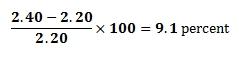lower than the 10% rate we saw for the previous year. This illustrates a key difference between linear and exponential growth — repeatedly increasing a quantity by a constant amount leads to smaller percent changes of the amount each time.

2. We compute 2.2×1.1=2.42. That is, a price increase of 22 cents would have had to occur in order to see an inflation rate of 10%.

The analogous comment to that in the previous part can be stated as follows — a constant annual percentage increase to leads to larger and larger annual increases in the cost of gasoline.

3. As we began to see in part (a), due to the compounding nature of exponential growth, a constant rate of inflation leads to ever-increasing price differences from one year to the next. The constant rate r would have to be less than 15%, since a 15% inflation rate for 8 consecutive years would increase the price by at least 15% of the starting price eight times, for a net gain of over 8×.15=1.2 times the starting price. That is, the initial price after eight years would have increased by 120%, giving a final price over double the initial price.

Perhaps surprisingly, we did not need to know either the starting or finishing prices in order to makes this line of reasoning, only their ratio (which, in this case of doubling, was simply 2).

4. We model the price of gasoline as a function of time: If A is the function that returns the price after n years of compounding, we have the standard annual compounding formula for A:where P is the initial amount or value and r represents the annual growth rate in the price of gasoline. In the case of doubling, the ratio of the final amount to the initial amount is 2 to 1, and so the given information about gasoline prices is the statement that A(8)=2P. Substituting this in the compounding formula gives 2P=P(1+r)8, or after dividing both sides by P, that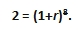One approach to solving this for r is to take the natural logarithm of both sides of the equation and to use properties of logarithms to re-write the expression:Dividing, we findExponentiating both sides to a base of e gives 1+r ≈ 1.0905, and so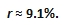5. The following table ftp://ftp.bls.gov/pub/special.requests/cpi/cpiai.txt gives annual CPI data for 1913 to 2012 (through the 3rd quarter). we record some observations before we being our calculations: Average annual increases in the CPI varied between 0.1 and 4.1 percent over the period 2004-2012. The average annual CPI for 2004 was 188.9 and for 2012 (through the first 3 quarters) was 231.32. Finally, the average annual increase in the CPI over this period can be roughly estimated by using the same solution method as we did above.

We can now repeat our calculations form the gasoline example, where instead of doubling over the 8-year span we have a ratio of 231.32/188.90. Setting up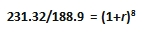and solving for r as above gives 1.025646 = 1+r, which gives an approximate average annual inflation rate of r≈2.6% over the period from 2004-2012. This is just over a quarter of the average annual rate of growth in the price of a gallon of regular gasoline (9.1%) over the same period in time, suggesting that something other than generic inflation is responsible for the tremendous rate of growth of the price of gasoline.

## SOLUTION: ALTERNATE SOLUTION FOR PARTS (D)-(E)

One can avoid the use of logarithms in the solutions to parts (d)-(e) as follows: Given 2=(1+r)8, we take the eighth roots of both sides and subtract 1 to get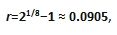or again r ≈ 9.1%.

For (E), the analogous calculation gives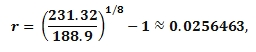so r≈2.6%.